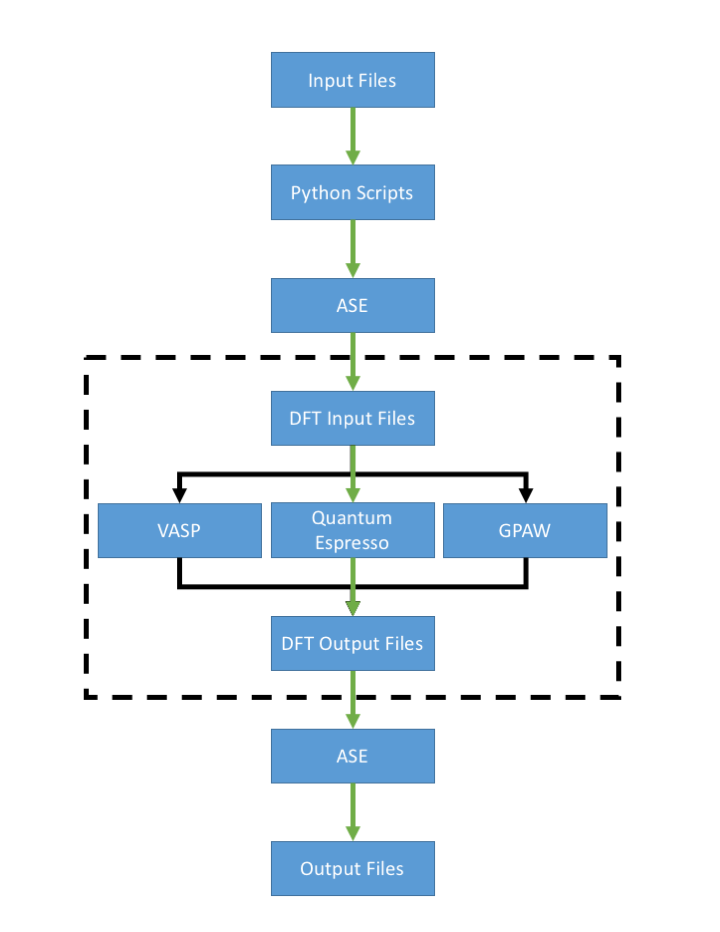# Background on our Calculations

This page attempts to deconvolute the different methods used in the group to perform Density Functional Theory (DFT) calculations. The different pathways for DFT calculations are shown in the flow chart below. The green line represents the most common path used in the group. Most members use the Atomic Simulation Environment (ASE) interfaced with the Quantum Espresso calculator to perform DFT calculations. The dashed box represents what is done when using "native" mode, bypassing any use of ASE.

• Input files
• ASE trajectories, eg init.traj
• Python scripts
• See the example scripts page.
• ASE
• See the ASE documentation here for ASE modules.
• DFT input files
• Quantum Espresso uses a file called pw.inp which can be found in the output directory, eg 'outdir/' or 'calcdir/'
• VASP uses several files:
• POSCAR contains the coordinates of all atoms in the unit cell, as well as information about the unit cell geometry. For MD calculations, it can also contain initial velocities.
• KPOINTS describes the k-point grid used to sample the Brillouin zone.
• POTCAR contains the pseudopotential used to describe the core electrons of each atom type in the unit cell.
• INCAR contains optional parameters used in the DFT calculation. See below for some of these parameters.
• GPAW uses ..
• Vienna Ab-Initio Simulation Package (VASP), Quantum Espresso, and Grid-based Projector Augmented Wave (GPAW) are different DFT calculators that can be used to calculate energies and forces of periodic systems.